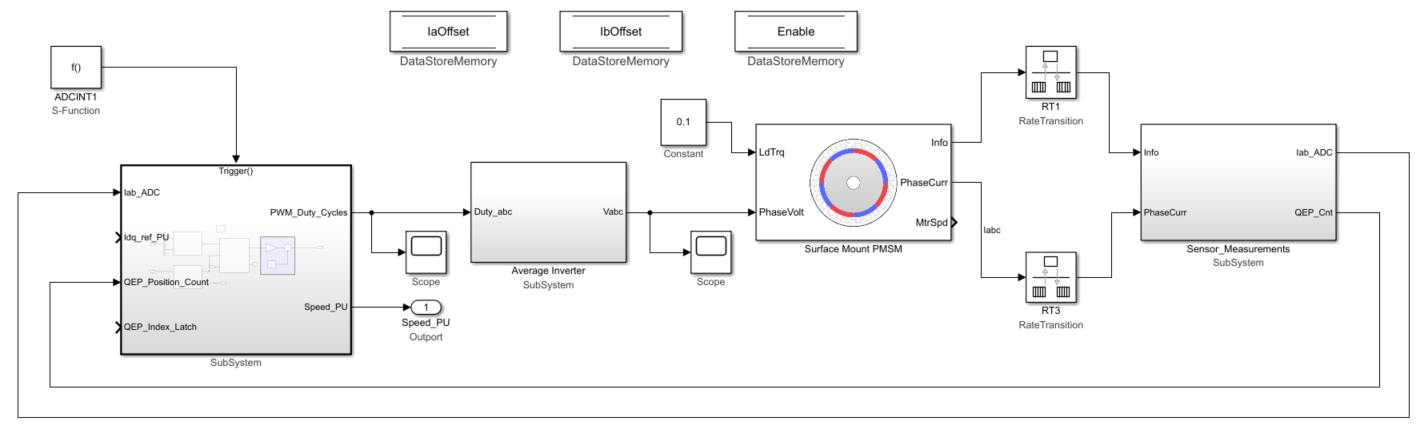## Design Current Controller Subsystem

Use these steps to design the current controller subsystem:

1. From the Motor Control Blockset™ library in the Simulink® Library Browser, use the Discrete PI Controller with anti-windup & reset block (in the Controls/Controllers library) to design the d-axis and q-axis current control. For example, this image shows the d-axis current controller subsystem.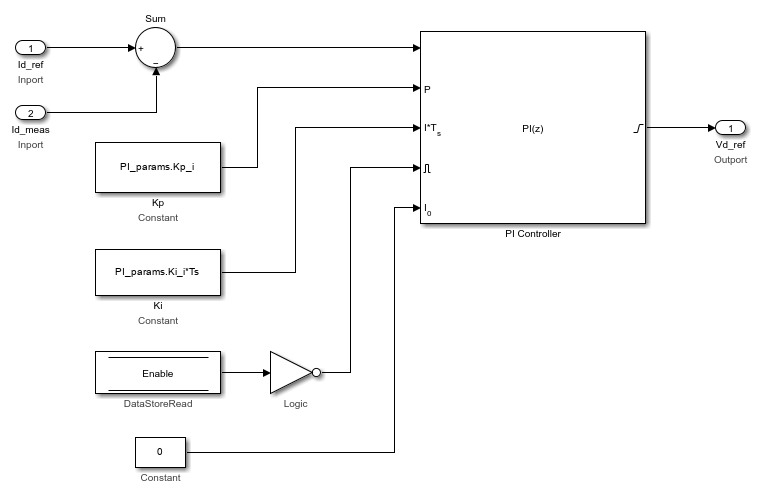The MATLAB® function `mcb.internal.SetControllerParameters` (in the model initialization script) calculates the PI control gains for the d-axis and q-axis current controller and the speed controller. For details about calculation of the controller gains, see Estimate Control Gains and Use Utility Functions. For example, see the model initialization script file `mcb_pmsm_foc_qep_f28379d_data.m` (used in the example Field-Oriented Control of PMSM Using Quadrature Encoder) that uses a sampling time (Ts) of `50` μs.

In the subsystem diagram, the Enable variable is a Data Store Memory used to reset the controller. Adding Enable variable is optional.

The subsystem also uses three constant blocks with these values:

• PI_params.Kp_i

• PI_params.Ki_i*Ts

• 0

Create a similar subsystem for the q-axis current PI controller. Integrate the subsystems for d-axis and q-axis PI controllers into a single subsystem (Current_Controllers) that controls the d-axis and q-axis currents.

2. Add the Clarke Transform, Park Transform, Inverse Park Transform, and Space Vector Generator blocks from the ```Motor Control Blockset/Controls/Math Transforms``` library to the Current_Controllers subsystem (that you created in step 1) as shown in this figure.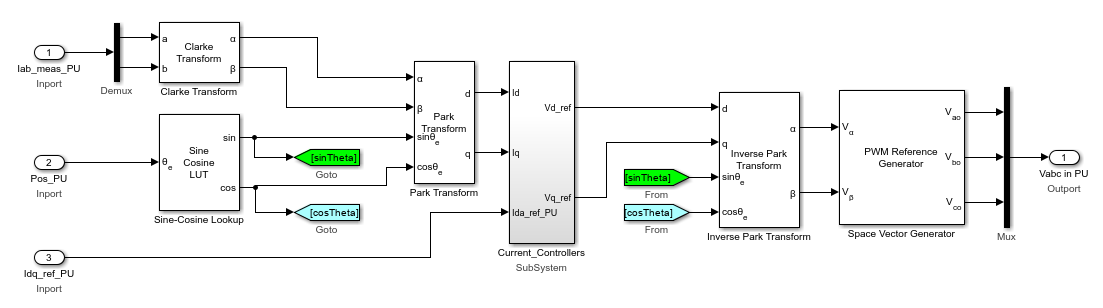3. Integrate the components that you created in step 2 into a single subsystem (Closed Loop Control that implements closed loop field-oriented control) as shown in this figure.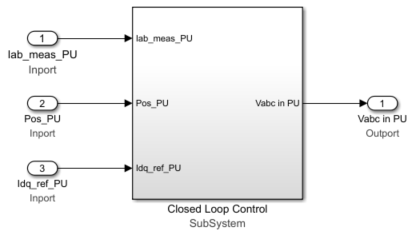4. Create an Output Scaling subsystem to scale the Pulse Width Modulation (PWM) outputs. This subsystem outputs the normalized PWM duty cycles (`0`-`1`) for the plant model.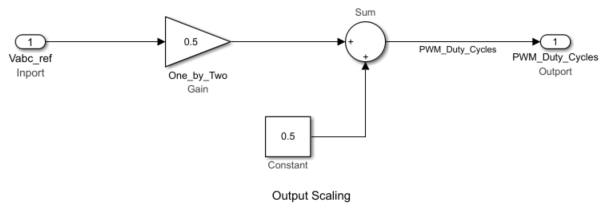5. Create a new subsystem by integrating the current scaling (Calculate Phase Currents), QEP position decoding (Calculate Position and Speed), Closed Loop Control, and Output Scaling subsystems. Add the Trigger block from the `Simulink/Ports & Subsystems` library to this subsystem and set the Trigger type parameter to `function-call`.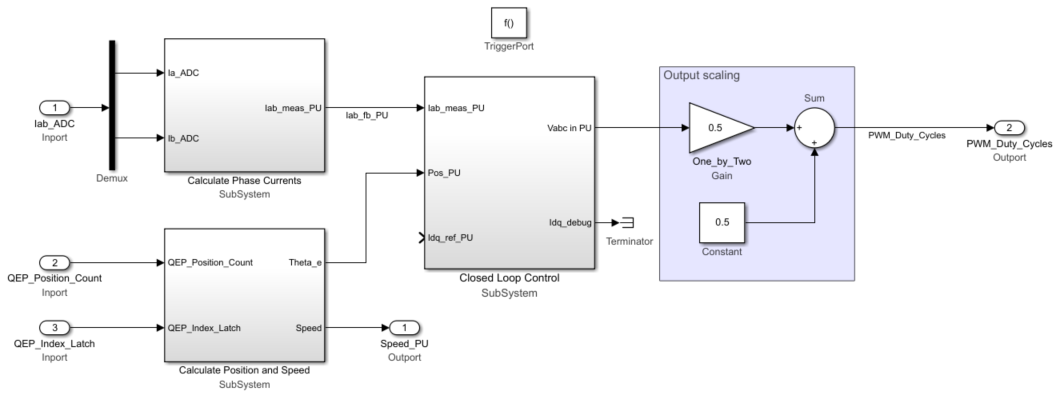6. Add a Function-Call Generator block from the `Simulink/Ports & Subsystems` library to the subsystem created in step 5. Set the Sample time parameter of the block to equal the control-loop sample time, Ts (that has a default value of `50e-6` s).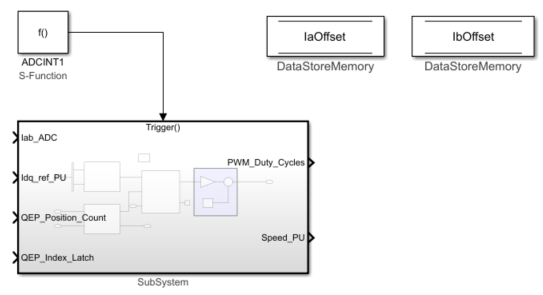7. Integrate the plant model and the controller subsystem that you created in step 6. For detailed steps on how to create a plant model for a motor control system, see Creating Plant Model Using Motor Control Blockset.